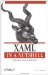# Point3D

 Point3D Point3D is a structure and has no class hierarchy.

`     <Point X="50" Y="100" Z="50" /> `

<Point3D .../> describes a point in x-, y-, and z-coordinates. Point3D is used primarily to describe more complex 3-D shapes.

Point3D is often declared through the use of abbreviated markup syntax. Wherever an attribute is specified as a Point, it can be declared as a comma-separated triplet of Double values representing the x-coordinate, y-coordinate, and z-coordinate, respectively:

` <Element SomePoint3DAttribute="0,0,0" /> `

#### Attributes

X (required)

A Double value representing the x-coordinate of the point

Y (required)

A Double value representing the y-coordinate of the point

Z (required)

A Double value representing the z-coordinate of the pointXAML in a Nutshell (In a Nutshell (OReilly))
ISBN: 0596526733
EAN: 2147483647
Year: 2007
Pages: 217